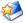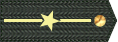# [原创]研制12至20吨级的混电重型通讯卫星意义极其深远

20吨级的通讯卫星末来可以使用 BFR来进行发射。倒是 12吨级的重型通讯卫星非常值得加快发展。因为长五、欧米加、火神、新艾伦、猎鹰 9H、长五 H与联盟 5的运力都在此一区间大量云集堆积，这非常有利于通讯卫星运营商来压低发射的价钱。因此未来 12吨级的混电通讯卫星的市场注定非常广阔，而且欧洲的阿里安六与日本的 H3火箭再努力下也能够参与这一区间载荷的发射竞争。还有如果 ULA的火神 542火箭的 GTO运力一旦能够维持于 1 3亿美元之内，并且 GTO运力也能够达到 12吨的话，对猎鹰 9H火箭的威胁还是很大的。

12吨的混电通讯卫星市场价格至少达 7 8亿美元一枚。而猎鹰 9H火箭的全复用版本的市场价格什么也要 9000万美元左右。遇上加州卫讯这样大土豪，运载火箭发射成本相差那 2000多万美元并不会成为左右火箭型号选择的最重要因素。但如果相差 5000万美元就有问题了。

打赏
收藏文本
0
0
2018/11/12 15:25:58

## 网友回复

•• 军号：3936821
• 工分:5632
左箭头-小图标

50吨级别，高轨道地球同步数字通讯卫星，天线直径为现在地球同步卫星的十倍，功率为现在的十倍。

土星5火箭发射是没有问题的，可惜美国人放弃了。

2018/11/13 15:15:52
左箭头-小图标

[size=10.5]12吨的混电通讯卫星相当于至少[size=10.5]7吨的全电通讯卫星了。而且[size=10.5]6[size=10.5]7亿美元的卫星，[size=10.5]6个月的利息支出也是很大的一笔成本，再加上[size=10.5]6个月时间的卫星折旧，长达[size=10.5]6个月的电推转移时间内的统合损失己高达[size=10.5]4000万美元左右。因此加州卫讯公司就己经为全球作出了选择的现成案例，即完全没有必要为运载火箭的那区区三、四千万美元的差异而浪费宝贵的时间。[size=10.5]
[size=10.5]
洛马公司早前就公开设想要研制重达[size=10.5]10吨左右的[size=10.5]AEHF改进型军事战略通讯卫星，并计划要使用德尔塔[size=10.5]4H火箭来发射。重型宽带通讯卫星本身就具有强大的军事应用潜力，而且美国欧洲政府现在也大量向商业市场租用卫星宽带服务。因此这是另一个明显的寓军于民的领域。如果不是全电通讯卫星的出现，现在的全化学推进通讯卫星的重量早就超过[size=10.5]10吨级了。还有现在重型全电通讯卫星己经衰落了。原因就是上面提出的那些观点。但混电通讯卫星未来要做到[size=10.5]10[size=10.5]20吨级绝对是发展的大趋势。[size=10.5]
[size=10.5]
核心的原因就是军事的需求极大，而且[size=10.5]GEO轨道的遥远距离也大大增加了卫星自身的安全性。至少美国军方现在就非常看重[size=10.5]GEO通讯卫星，这也必然存在其他的深层原因。现在的[size=10.5]AEHF[size=10.5]MOUS军事通讯卫星的重量也接近[size=10.5]7吨了（都是混电通讯卫星），再增加一倍做到[size=10.5]14吨级也就只是时间的问题而己。[size=10.5]
[size=10.5]
象美国的[size=10.5]MOUS海事通讯卫星，只要那个可展开式天线换成大酒瓶电子侦察卫星的那个直径[size=10.5]150米级的超级天线。总重量就接近[size=10.5]10吨了。在小型战术无人飞机正在爆炸性发展扩散的今时今日，[size=10.5]MOUS卫星那怕做到[size=10.5]20吨级都没有人会嫌大的。[size=10.5]
[size=10.5]
缩小无人机卫星通讯天线的口径，并减少设备的电力消耗，个人手持终端也是如此。[size=10.5]4G手机的天线能够做到那么小，是因基站天线就在附近，联接距离并不远。但[size=10.5]GEO卫星与地面的距离已经固定，只要通讯卫星越来越大，地面的卫星手机天线就可以越做越小。最后[size=10.5]GEO通讯卫星做到[size=10.5]50[size=10.5]100吨，天线口径做到[size=10.5]300米、甚至[size=10.5]500米，地面卫星手机的天线与功耗就能够与今天的[size=10.5]4G手机越来越趋同一体化了。因此[size=10.5]GEO轨道通讯卫星的增大趋势远远末到尽头之时，大酒瓶电子侦察卫星的[size=10.5]150米级天线被最终整合到民用移动通讯卫星上面也就只是时间迟早的问题。目前民用版本的移动通讯卫星的可展开式天线最大直径的才不过[size=10.5]36米（一说是[size=10.5]48米，由诺格公司生产），这离大酒瓶卫星的[size=10.5]150米还相差极远。[size=10.5]
[size=10.5]
粗略估计下，如果用上[size=10.5]1具这种[size=10.5]150米口径的天线研制巨型多波束移动通讯卫星的接收天线，整颗通讯卫星的重量至少要达[size=10.5]10吨以上。如果再升级到[size=10.5]300米直径接收天线，整颗通讯卫星的重量什么也要提升到[size=10.5]15[size=10.5]20吨的级别。因此，即使以现在的技术水平，移动通讯卫星与宽带通讯卫星也完全能够做到[size=10.5]15[size=10.5]20吨级了。其实那个[size=10.5]NRHO深空站的核心舱就是未来巨型通讯卫星极好的推进舱平台，电力供应也有[size=10.5]40[size=10.5]50Kw，重量也才不过[size=10.5]7[size=10.5]5吨，如果基于这个平台做个[size=10.5]15[size=10.5]20吨级的巨型通讯卫星，再使用[size=10.5]SLS火箭（或者[size=10.5]BFR）直接送入[size=10.5]GEO轨道。[size=10.5]SLS火箭末来也饿不死了。[size=10.5]
[size=10.5]
以后无论是中国还是美国，都完全可以基于各自的[size=10.5]NRHO深空站的推进舱为核心研制自己的[size=10.5]GEO通讯空间站，每个[size=10.5]GEO空间站都重达[size=10.5]80吨级，由[size=10.5]4[size=10.5]5个舱段平台所组成。平时无人看管，但可按时发射货运飞船补加燃料，坏了也可以派遣载人飞船前去进行人工修理。三个[size=10.5]GEO深空站即可满足一个国家除两极地区之外的全球绝大部分的通信需求了。而且每个通讯空间站的寿命都可长达[size=10.5]30年。[size=10.5]
[size=10.5]
载人飞船能够前住[size=10.5]NRHO深空站，也一样能够前往[size=10.5]GEO通讯空间站。可维护的[size=10.5]GEO通讯空间站实际上就极象今天地球上的[size=10.5]4G通讯基站。因为也一样能够进行人工的定期维护管理。[size=10.5]
[size=10.5]
总结，高凉陈君认为搞这个[size=10.5]GEO通讯空间站可比搞什么太空发电站要靠谱得多。而且使用中间运力型火箭就可以直接建设。[size=10.5]
[size=10.5]
建设[size=10.5]GEO通讯空间站最大的优势就是后期可进行人工维护管理，而且电力也可以做得非常之大，达[size=10.5]100[size=10.5]200Kw都可以。能够建设[size=10.5]NRHO深空站的国家都可以建设与进行日常维护。而且还能够真正赚钱，因此这个东西一旦上马建设，也真正为中间运力型火箭（乃至巨型火箭）找到了一张可靠的长期饭票。这叫统等发展，协同进步。国营的卫星通讯公司承担一下国家义务没什么事情。反正政府机构也是国营卫星公司的使用大客户。至于民营卫星通讯公司就“自便”了。[size=10.5]
[size=10.5]
如果这个[size=10.5]GEO通讯空间站的供电功率规模再增加[size=10.5]10倍，达到[size=10.5]1000Kw[size=10.5]2000Kw的水平，就逐渐具备了布置天基激光器的潜力（对付洲际导弹与卫星）了。这个才是真正的星球大战杀手锏。[size=10.5]
陈天（高凉陈君）

20181111

2018/11/12 15:48:26
左箭头-小图标

四，通过上面粗略的建模分析，在发射[size=10.5]GTO12吨以上的重型载荷时，火神火箭相对于猎鹰[size=10.5]9H火箭的价格劣势将大大缩小。如果[size=10.5]GTO运力上升到[size=10.5]16[size=10.5]22吨区间，猎鹰[size=10.5]9H相对于火神火箭的价格优势则越来越小，没有更大直径的整流罩可使用的劣势也越来越大。而火神火箭与长征五号[size=10.5]H型火箭的整流罩直径要升级到[size=10.5]6米以上，则完全是一件易如反掌的事情。因此洛马、波音与长城工业公司未来不研制重达[size=10.5]12[size=10.5]20吨级的混电通讯卫星才真正是与自己的火箭产业过不去。[size=10.5]
[size=10.5]
这个分析列表对阿里安航天、[size=10.5]ULA、三菱重工与长城工业公司都具有重要的参考意义。一次性火箭要想在猎鹰[size=10.5]9[size=10.5]9H可重复使用火箭的阴影下长久地生存下去，开发[size=10.5]12[size=10.5]20吨区间的重型混电通讯卫星绝对是唯一的出路了。[size=10.5]
[size=10.5]
如果[size=10.5]12吨级的混电通讯卫星能够控制于[size=10.5]6亿美元一枚，[size=10.5]20吨级的混电通讯卫星能够控制于[size=10.5]10亿美元一枚。未来波音、洛马与长城工业公司是一定会研制的，因为这是保住他们自己的一次性火箭生产线的最后机会。如果火神[size=10.5]562、联盟[size=10.5]5与长征五号[size=10.5]H火箭的市场价格也能够控制于[size=10.5]1[size=10.5]5亿美元（左右）一枚的区间，自然也就不必害怕猎鹰[size=10.5]9H火箭的竞争压力了。倒是欧洲的阿里安[size=10.5]6火箭与日本的[size=10.5]H3火箭才是真正的压力巨大。[size=10.5]
[size=10.5]
要发射[size=10.5]16[size=10.5]20吨级别的混电通讯卫星，猎鹰[size=10.5]9H火箭的可重复使用优势就通通被废掉了，新艾伦火箭也一样如此。因为一款火箭的最佳复用运力区间都是有限的，这就涉及到如何进行合理的取舍问题了。而目前全球通讯卫星市场研制[size=10.5]10[size=10.5]12吨区间的重型混电通讯卫星的时机己经完全成熟。如美国卫讯公司的那三颗重型宽带通讯卫星，质量做到[size=10.5]9吨的区间才明显具有运营与发射的最佳经济合理性。反正都已经决定要采用“包箭发射”的方案了，卫星现在才重[size=10.5]6[size=10.5]7吨，这样显然就白白浪费了近[size=10.5]2[size=10.5]3吨的火箭运力。[size=10.5]
[size=10.5]
[size=10.5]12吨级的混电通讯卫星与[size=10.5]7吨级全电通讯卫星都按[size=10.5]6亿美元价格来计算，卫星寿命则以[size=10.5]15年来计算，平均每个月折旧损失[size=10.5]333万美元。推迟定位[size=10.5]4个月则损失[size=10.5]1300万，推迟[size=10.5]6个月定位则损失[size=10.5]2000万美元。还有，猎鹰[size=10.5]9火箭只能发射[size=10.5]7吨级别的混电通讯卫星，发射不了[size=10.5]7吨级的全电通讯卫星，否则在范艾伦带里转太久，卫星也要废的。即全电通讯卫星的入射轨道也是有门槛的，初始轨道太低也是不行的。因此卫讯公司的重型宽带通讯卫星也是要用到猎鹰[size=10.5]9H火箭来发射。[size=10.5]
[size=10.5]
还有高凉陈君一直是坚定支持可重复使用火箭的，本文的本意是为了说明在特定的运力区间，一次性火箭还是可以一战的。[size=10.5]
[size=10.5]
别说[size=10.5]7吨的全电通讯卫星要至少[size=10.5]6[size=10.5]7亿美元一颗，[size=10.5]7吨级别的混电通讯卫星现在也很昂贵。长征五号遥[size=10.5]2火箭所发射的那枚东方红[size=10.5]5号试验通讯卫星的质量就是[size=10.5]7吨左右，价格外界传言高达[size=10.5]50亿人民币（即已经高达[size=10.5]7亿多美元一枚）。而美国卫讯公司的卫讯三宽带通讯卫星发展计划，其中的每一颗卫星都要[size=10.5]5亿美元以上。因此末来那些重达[size=10.5]12[size=10.5]20吨级别的巨型通讯卫星，什么也要[size=10.5]8[size=10.5]15亿美元一颗的。此时运载火箭的价格就不是唯一的考虑条件了，发射的高可靠性才是更重要的选择因素。现在卫讯公司在发射卫讯三时就没有过多扣扣索索火箭的价格高低问题。连[size=10.5]ULA公司市场价格接近[size=10.5]2亿美元一枚的宇宙神[size=10.5]551火箭都敢使用，可见卫讯公司对火箭的可靠性有多么的看重。也从侧面可知卫讯三系列卫星有多么的昂贵。坦率而言，重达[size=10.5]12吨级别的混电商业通讯卫星，没有[size=10.5]7[size=10.5]8亿美元是拿不下来的，这种级别的卫星就是使用德尔塔[size=10.5]4H火箭来发射也是值得的。[size=10.5]
[size=10.5]
做大生意就必须要有大魄力，卫讯公司就是如此。[size=10.5]
陈天（高凉陈君）

20181110

第二节 运营火箭需要严肃认真的负责任态度。
每一款火箭在其整个使用生涯之中累积的发射量如果太少的话，巨额的研制成本是根本无法摊薄下来的。[size=10.5]

回到[size=10.5]SLS火箭的身上，[size=10.5]SLS火箭的整个研制成本现在已经接近[size=10.5]100亿美元。如果整个生命周期的发射量为[size=10.5]50枚，则每一枚火箭的均摊研制成本为[size=10.5]2亿美元；但如果发射量为[size=10.5]500枚的话，每枚火箭的均摊研制成本就已经下降到[size=10.5]2000万美元了。如阿波罗时代的质子火箭到今天为止的发射总量已经接近[size=10.5]400枚（至于阿波罗时代的联盟系列火箭到今天为止，其的发射总量更是超过了[size=10.5]1800枚），但美国同时代同等运力水平的土星[size=10.5]1B火箭的发射总量却只有不足[size=10.5]20枚。[size=10.5]

[size=10.5]
因此，那怕今天的美国也依旧富有，但在研制与运营[size=10.5]SLS火箭时，白宫与国会内部也一样是指责声音不断。从长远角度而言，质子与联盟火箭的运营态度还是存在重大的借鉴参考价值的。毕竟人类的火箭工业要进入良性循环的“可持续发展道路”，学习[size=10.5]NASA运营土星[size=10.5]1B、土星[size=10.5]5[size=10.5]SLS火箭的态度绝对是死路一条。否则那怕有金山银山最后也会吃空、吃垮的。
第三节 研制通讯空间站的时机已经成熟。
火神火箭的最终运力还没能确定，但[size=10.5]GTO运力推测能够达到[size=10.5]15吨区间。否则如何取代德尔塔[size=10.5]4H火箭？！还有高价值载荷（指单价[size=10.5]6[size=10.5]7亿美元以上的载荷）对相差[size=10.5]2000[size=10.5]3000万美元的火箭价格差异并不敏感。对于猎鹰[size=10.5]9[size=10.5]9H火箭而言，其运力最佳的优化区间是[size=10.5]GTO12吨以下的区域，这就为一次性火箭留下了一块生存的自留地了，也仅此而己。[size=10.5]
[size=10.5]
其实火神火箭未来商业市场的竞争力主要取决于固体燃料助推器的市场价值了。如果诺格公司不肯降价，[size=10.5]ULA公司也无能为力，只能死抱政府任务就了事。但如果火神[size=10.5]562的市场价格能降低到[size=10.5]1[size=10.5]3亿美元左右，其之商业市场的竞争力还是非常强大的。当然这也要波音、洛马公司肯主动去配合，如果波音洛马不肯去主动研制[size=10.5]12吨级的混电通讯卫星，那么[size=10.5]ULA也无可奈何。[size=10.5]
[size=10.5]
高通量宽带通讯卫星做到[size=10.5]12[size=10.5]20吨级的可能性是完全存在的，通讯卫星两极分化是一个非常明显的发展趋势。而且高价值卫星也全部是走大单星的包箭发射方案，因此也非常有利于通讯卫星越做越大。对于卫讯、海事卫星组织、国际卫星组织、欧洲卫星组织等公司而言。运营[size=10.5]12吨级的混电通讯卫星在客户开拓、金融信贷支持等方面也不存在不可克服的困难。至于洛马与波音，只要将目前基于[size=10.5]A2100[size=10.5]BSS702平台而研制的[size=10.5]AEHF[size=10.5]MOUS[size=10.5]WGS军用通讯卫星改进升级一下，就可以迅速研制出[size=10.5]12吨级别的民用重型宽带通讯卫星来。[size=10.5]
[size=10.5]

2018/11/12 15:37:20

总页数11页 [共有4条记录] 分页：

1
对[原创]研制12至20吨级的混电重型通讯卫星意义极其深远回复